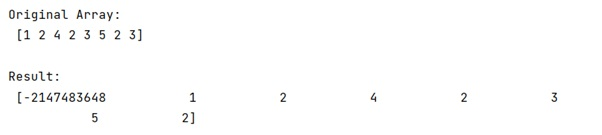# Shift elements in a NumPy array

Learn, how to shift elements in a NumPy array in Python?
Submitted by Pranit Sharma, on January 06, 2023

NumPy is an abbreviated form of Numerical Python. It is used for different types of scientific operations in python. Numpy is a vast library in python which is used for almost every kind of scientific or mathematical operation. It is itself an array which is a collection of various methods and functions for processing the arrays.

## NumPy Array - Shifting elements

Shifting an array includes removing the elements from an array and returning the removed elements. This method changes the length of the array.

If we want to shift the elements of a NumPy array, we need to use the shift() function from scipy library where the default is to bring in a constant value from outside the array with value cval, set here to nan.

Let us understand with the help of an example,

## Python code to shift elements in a NumPy array

```# Import numpy
import numpy as np

# Import shift method
from scipy.ndimage.interpolation import shift

# Creating an array
arr = np.array([1,2,4,2,3,5,2,3])

# Display original array
print("Original Array:\n",arr,"\n")

# Shifting the elements of the array by one
res = shift(arr, 1, cval=np.NaN)

# Display result
print("Result:\n",res,"\n")
```

Output: